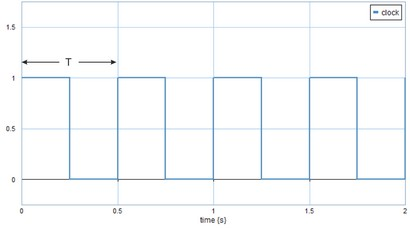﻿ 20-sim webhelp > Library > Signal > Logical > Boolean > Clock

# Clock

## Library

Signal\Logical\Boolean

## Use

Domains: Discrete, Continuous. Size: 1-D. Kind: Block Diagrams.

## Implementations

This model has a continuous-time and a discrete-time implementation.

 • Continuous
 • Discrete

## Description

This models generates a logical continuous clock signal, i.e. a signal that changes from true (1) to false (0) and back, each period T with T = (1/frequency)If the discrete implementation is chosen, the frequency must be smaller than half of the  the sample frequency, otherwise not a good clock signal will result. To have the top part of the clock signal equally long to the down part, choose the frequency equal to the sample frequency divided by 2^n, with n an integer equal or larger than one.

## Interface

 Port name Data type Description Unit Range Parameters initial boolean initial output false/true frequency real clock frequency Hz > 0.0 Hz Outputs output boolean Clock output false/true

## Restrictions

Make sure that the simulation uses step sizes which are small enough to calculate the clock signal going up and going down: step size << clock period.